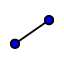# Medians of a Triangle

Use the midpointtool to find the midpoints of the three sides of the triangle.

Did you find the midpoints of the three sides?

Select all that apply
• A
• B
Use the segment toolto connect each midpoint to the opposite vertex, constructing the medians of the triangles.

Did you draw the three medians of the triangle?

Select all that apply
• A
• B

What do you notice about where the medians intersect?

## Move the vertices of the triangle around.

What happens to the point of intersection of the medians?

The centroid is the point of intersection of the three medians of a triangle. Write this in your notes!
Place a point at the centroid of the triangle using the intersectiontool.

Can the centroid ever be located outside the triangle? Move the vertices around and see what happens before answering?

Select all that apply
• A
• B

Did you place a point at the centroid?

Select all that apply
• A
• B
Use the distance measurement toolto measure the lengths of the portions of the median from the vertex to the centroid, and then from the centroid to the opposite side. You should end up with 6 different lengths labeled on the diagram.

Do you have 6 segment lengths?

Select all that apply
• A
• B

## Move the vertices of the triangle and observe the lengths of the segments you measured.

What is the relationship between the distance from a vertex to the centroid and the distance the rest of the way from the centroid to the opposite side? If you don't see it immediately, try manipulating the vertices so that at least one of the lengths is an integer.